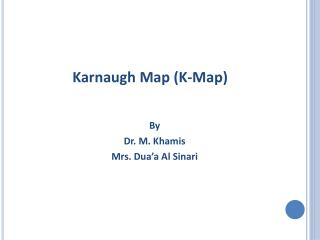DownloadDownload PresentationKarnaugh Map (K-Map)

# Karnaugh Map (K-Map)

Download Presentation## Karnaugh Map (K-Map)

- - - - - - - - - - - - - - - - - - - - - - - - - - - E N D - - - - - - - - - - - - - - - - - - - - - - - - - - -
##### Presentation Transcript

1. Karnaugh Map (K-Map) By Dr. M. Khamis Mrs. Dua’a Al Sinari

2. Continue logic gates Operation: NAND (the complement of the product) NOR (the complement of the sum) XOR (Exclusive OR) F=(xy)’, or F=(xy)’ F=xy’+ x’y Expression: F=(x + y)’ Number Systems and Boolean Algebra Logic gate: Truth Table:

3. Gate-Level Minimization • The complexity of the digital logic gates is related to the complexity of the algebraic expression. • The map method provides a simple, straightforward procedure for minimizing Boolean functions. • The map method is also known as Karnaugh map or k-map. Two-variable Map: y Number Systems and Boolean Algebra y 0 1 X 0 1 X m1 +m2 +m3 =x’y + xy’ +xy = x+y

4. TWO-VARIABLE MAP: y y y y 0 1 0 1 X X 0 0 1 1 X X (b) x+y (a) xy Three-VARIABLE MAP: y yz 00 01 10 11 X 0 m5 + m7= xy’z+ xyz = xz(y’ + y) =xz 1 X z

5. EXAMPLE(1): Simplify the Boolean function F(x,y,z) = ∑ (2,3,4,5) x’y y m3 + m2 = x’yz + x’yz’=x’y(z+z’)=x’y m4 + m5 =.................??...............=xy’ F(x,y,z)=∑(2,3,4,5)= x’y + xy’ yz 00 01 10 11 X 0 1 X z xy’

6. training • Try this one: (simplify the Boolean function) • F(x,y,z) = ∑ (3,4,6,7) • Solution: after representing k-map the simplification will be as shown F= yz + xz’ • F(x,y,z) = ∑ (0,2,4,5,6) • Solution: after representing k-map the simplification will be as shown F= z’ + xy’

7. EXAMPLE(2): • Let the Boolean function F= A’C + A’B + AB’C + BC A) Express this function as a sum of minterms. B) Find the minimal sum-of-products expression. Solution: A) F(A,B,C)= ∑(1,2,3,5,7) B) F= C + A’B B BC 00 01 10 11 A 0 1 A C

8. Four-variable map: Y YZ 00 01 10 11 WX 00 01 X 11 W 10 Z

9. Facts for simplification: • The combination of adjacent squares that is useful during the simplification process is easily determined from inspection of the four-variable map: • One square represents a term with four literals. • Two adjacent squares represent a term with three literals. • Four adjacent squares represent a term with two literals. • Eight adjacent squares represent a term with one literal. • Sixteen adjacent squares produce a function that is always equal to 1.

10. Example(3): • Simplify the Boolean function F(w,x,y,z)=∑(0,1,2,4,5,6,8,9,12,13,14) w’y’z’ + w’yz’ =w’z’ xy’z’ + xyz’ =xz’ F(w,x,y,z)= y’+ w’z’+ xz’ Y w’yz’ YZ 00 01 10 11 WX 00 w’y’z’ 01 X 11 W 10 xyz’ xy’z’ Z Y’

11. Example(4): • Simplify the Boolean function: F=A’B’C’ +B’CD’ + A’BCD’ + AB’C’ TRY TO REPRESENT IT YOURSELF ON K-MAP Solution: F= B’D’ + B’C’ + A’CD’